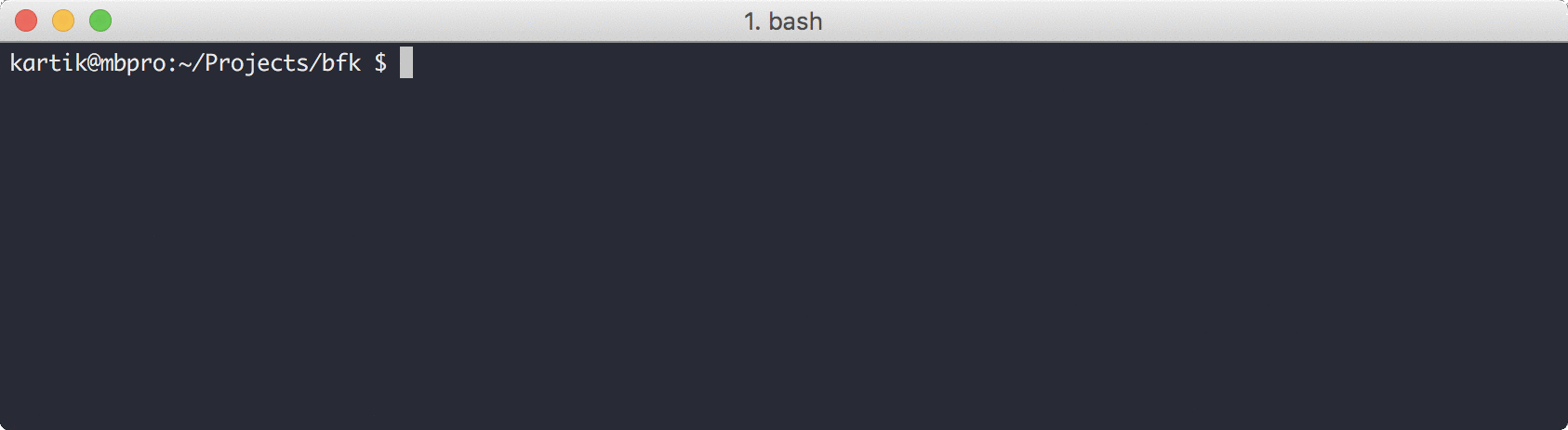### Writing a BrainF**k Interpreter

Recently while going through learxinyminutes I stumbled upon the (very) short documentation of BrainF**k programming language. I’ve known about this esoteric language for a long time but never took out time to read more about it. Looking into the documentation it seemed that the language has just a handful of commands and would be a great starting point for writing interpreters.

In this case the documentation itself spells out everything we need to create our own BrainF**k interpreter. I’ll be doing this in Python, but feel free to use any programming language you’re comfortable in. Making this interpreter could also help you in getting comfortable with the language you’re currently learning.

Copying directly from the learnxinyminutes website, here is the list of eight commands we need to handle:

``````+ : Increments the value at the current cell by one
- : Decrements the value at the current cell by one
> : Moves the data pointer to the next cell (cell on the right)
< : Moves the data pointer to the previous cell (cell on the left)
. : Prints the ASCII value at the current cell (i.e. 65 = 'A')
, : Reads a single input character into the current cell
[ : If the value at the current cell is zero, skips to the corresponding ] . Otherwise, move to the next instruction
] : If the value at the current cell is zero, move to the next instruction. Otherwise, move backwards in the instructions to the corresponding [
``````

The runtime also requires an array of `30000` cells all initialised to zero and a data pointer pointing towards the first cell of this array.

Apart from the data pointer, we’ll use another variable called program counter which will point towards the current instruction we need to execute, just like how the CPU operates.

Let’s start with the pointers and array, we’ll also create a variable named `program` which will accept the BrainF**k program as user input.

``````# initialize dc array with 30000 "0"s
data_array = *30000

# make a list of commands
program = input().strip()

# program counter
pc = 0

# data counter
dc = 0
``````

One more thing that we need, and what actually makes this interpreter something other than easy, is the loop stack. The square brackets `[` and `]` effectively represent while loops. One thing you would have noticed while looking at the definition for `]` is that it can jump towards the corresponding `[`. To be able to do this we need to save the position of `[`, so that we can set our program counter to it. But, we can have nested loops, and thus whenever we get a `]`, we need to jump to the last `[` we found. This can be achieved by using a stack data structure (python lists can be easily used as stacks).

``````# stack for storing loop counter
loop = []
``````

Now let’s actually start interpreting programs, our program variable string contains the individual commands we need to execute. Instead of iterating character by character through the program string, we’ll create an infinite while loop and iterate through the program manually through our `pc` variable. This is done because if we use an indexed for loop, it would become difficult to jump through instructions (Can be done, but not required). To break out of the program, we’ll just rely on catching the `IndexError` exception in Python which will tell us that we have effectively reached the end of program string.

``````# run forever
# we can have potential infinite loops
while (1):
try:
cmd = program[pc]
except IndexError:
# we have reached the end
break
``````

Now the only thing left is actually executing the commands, we’ll do this using a series of `if-elif` statements. Depending upon the command, we’ll either increase/decrease the data counter, or increase/decrease the actual cell pointed by the data counter. The two command we need to focus on are `[` and `]`, rest are pretty self-explanatory.

For the opening square bracket, we need to skip the loop if the current cell pointed to by the data pointer is zero, for achieving this we’ll just skip towards the closing square bracket using a while loop. If the value pointed to is not zero, we’ll store the current loop pointer and start executing the next instruction.

For the closing square bracket, if the current cell pointed to by the data pointer is not zero, then we have to jump to the corresponding opening bracket, this we’ll get from our loop stack, if not then we just continue with the next instruction (but we still need to pop the stack)

``````    if cmd == '+':
data_array[dc] += 1
elif cmd == '-':
data_array[dc] -= 1
elif cmd == '>':
dc += 1
elif cmd == '<':
dc -= 1
elif cmd == '.':
asc = chr(data_array[dc])
print (asc, end='', flush=True)
elif cmd == ',':
ch = input().strip()
data_array[dc] = ord(ch)
elif cmd == '[':
if data_array[dc] == 0:
while (program[pc] != ']'):
pc += 1
continue
else:
# store the loop program counter
# maintain a stack for nested loops
loop.append(pc)
if cmd == ']':
if data_array[dc] != 0:
pc = loop.pop()
continue
loop.pop()

# move to next command
pc += 1
``````

And that’s it. Here’s the whole program in its entirety :

And here’ the script running: